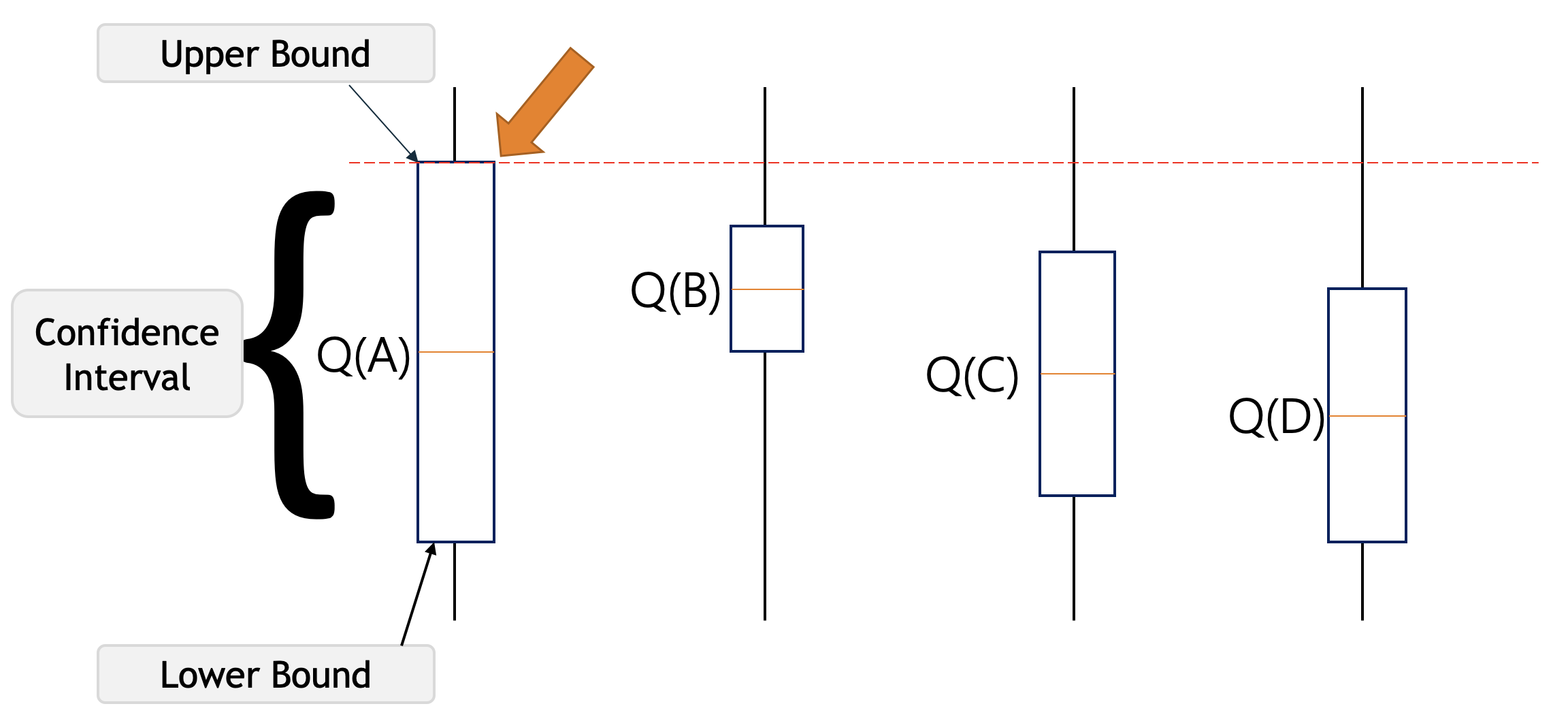# In UCB, is the actual upper bound an upper bound of an one-sided or two-sided confidence interval?

I'm a bit confused about the visualization of the upper bound (following the notation of (c.f. Sutton & Barto (2018))

$$Q_t(a)+C\sqrt{\frac{\mathrm{ln}(t)}{N_t(a)}}$$

In many blog posts about the UCB(1)-algorithm, such as visualized in the following image (c.f. Link ):Isn't the upper (confidence) bound simply the upper bound of a one-sided confidence interval instead of a two-sided confidence interval as shown in the image above? A lower bound of the interval is completely useless in this case, or am I wrong?

• As far as I am aware it is for a two sided confidence interval. I only have a brief knowledge of MAB's so I don't want to write an official answer but I am fairly certain a paper I read on them a few years ago also proved a lower bound (I guess for completeness) because you are right in saying that a lower bound would be useless for a MAB. – David Ireland Jan 19 at 21:08
• In the MAB domain, it is possible to specify a lower as well as an upper bound for the regret of an algorithm. Probably you mix it up with that? – D. B. Jan 19 at 21:39